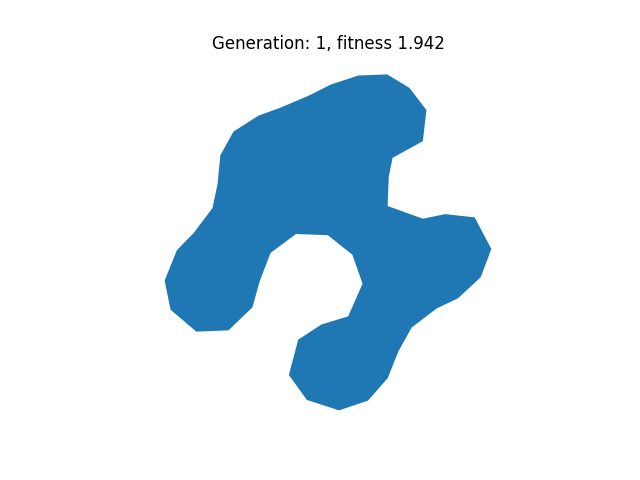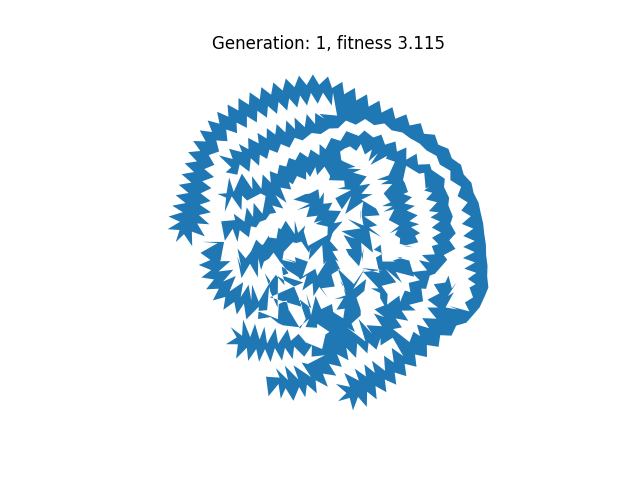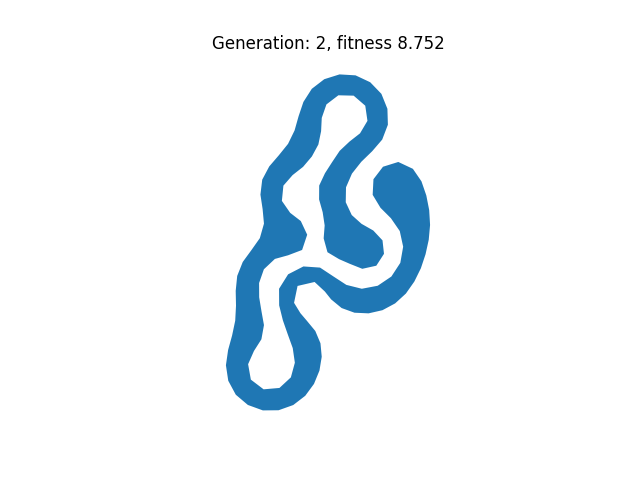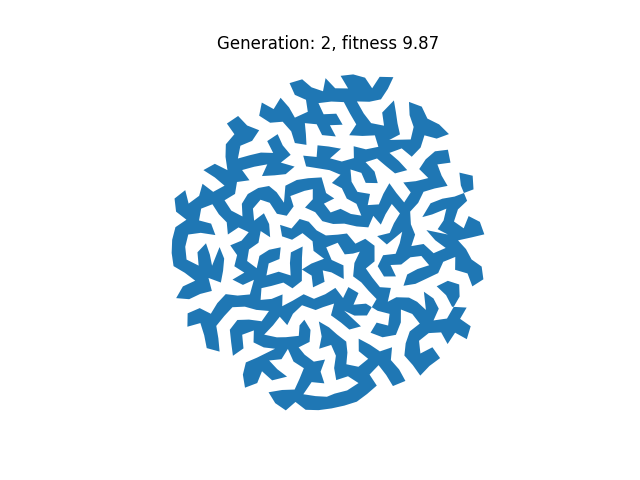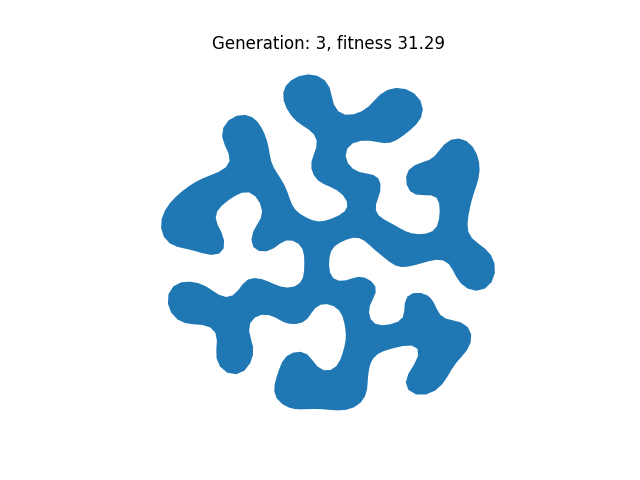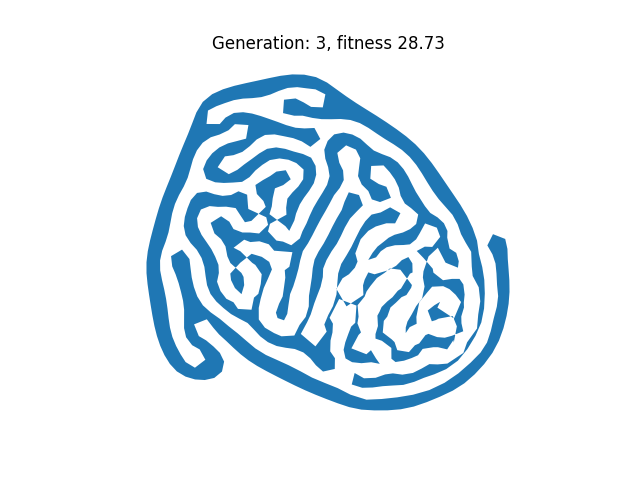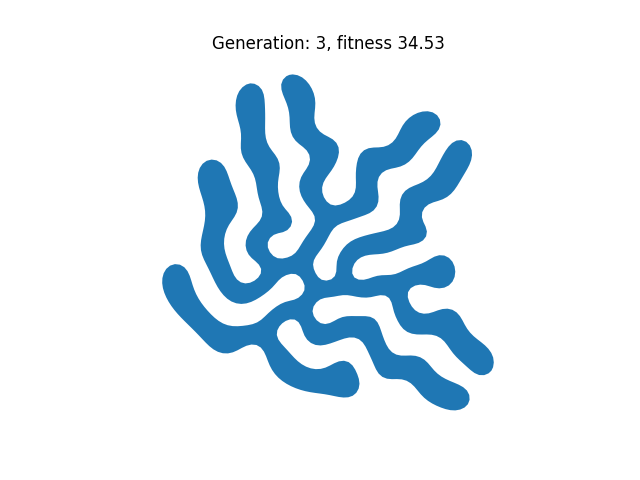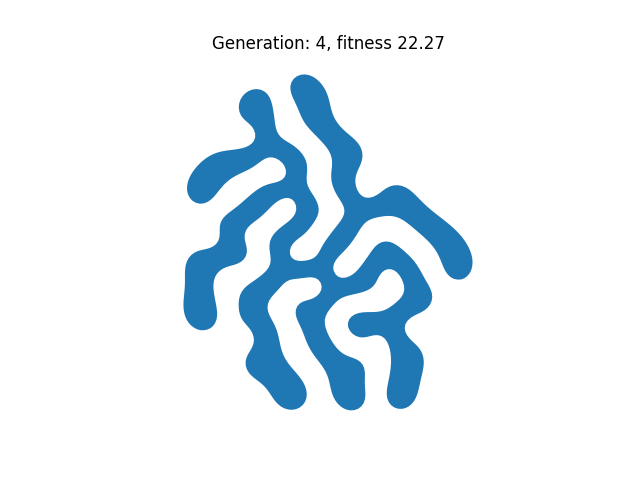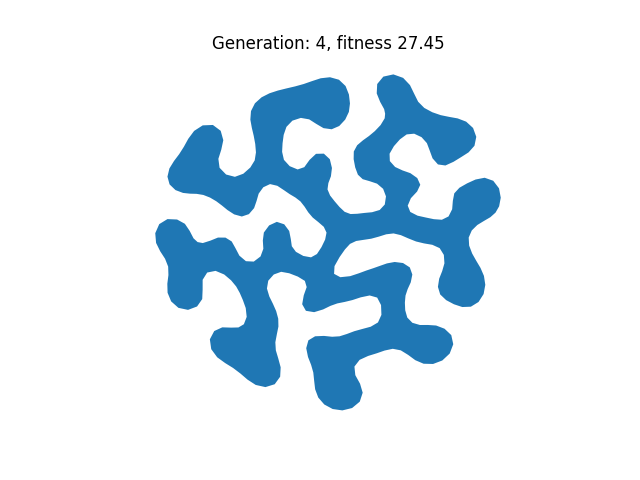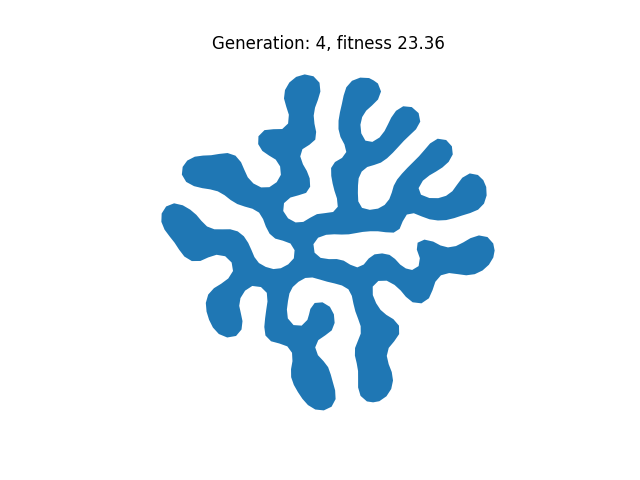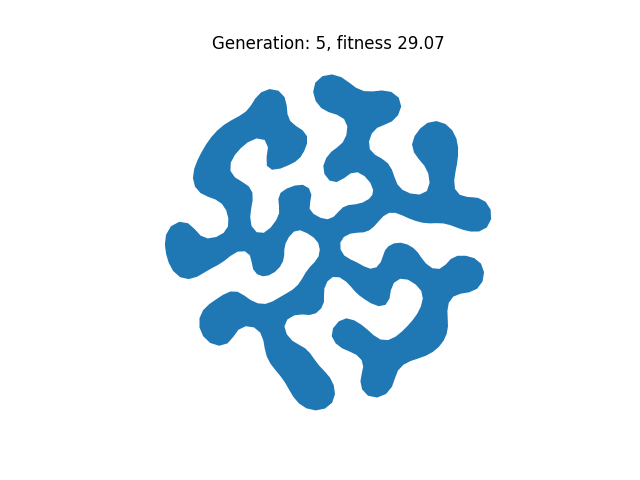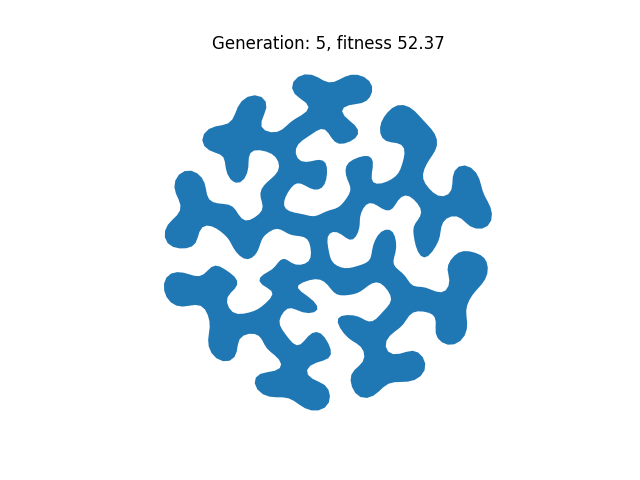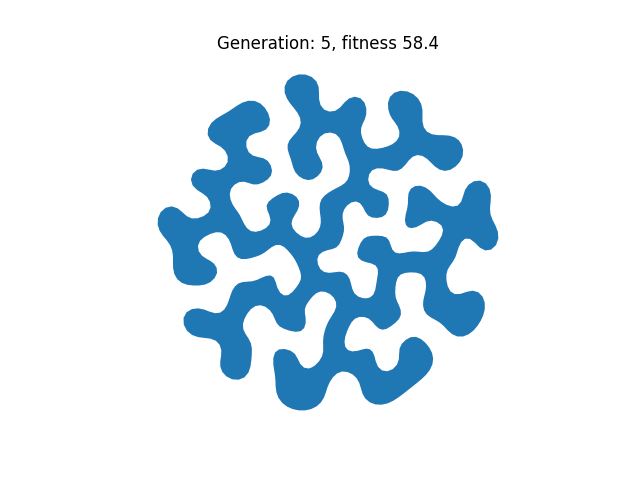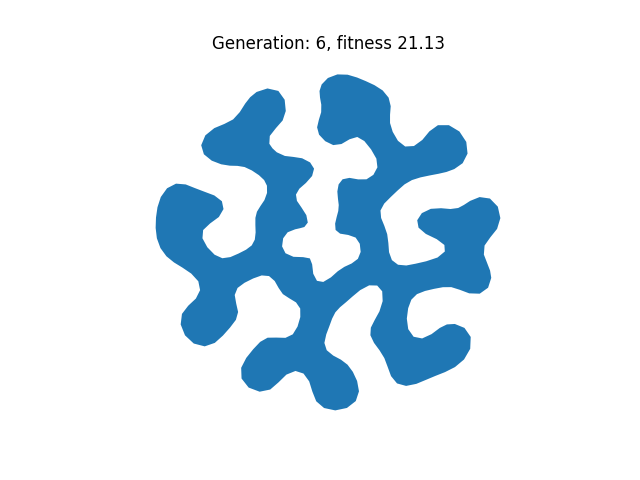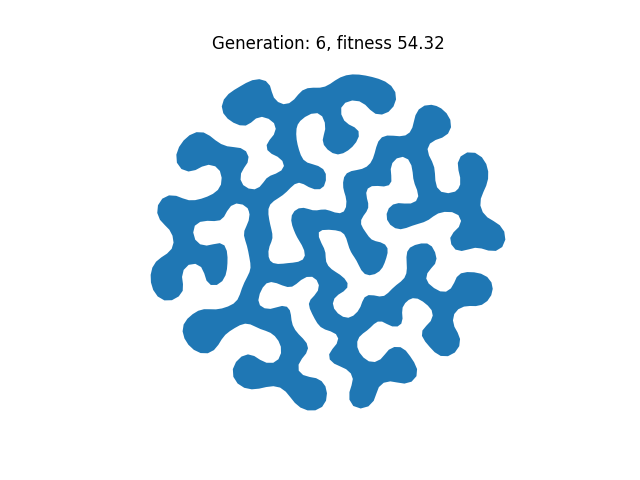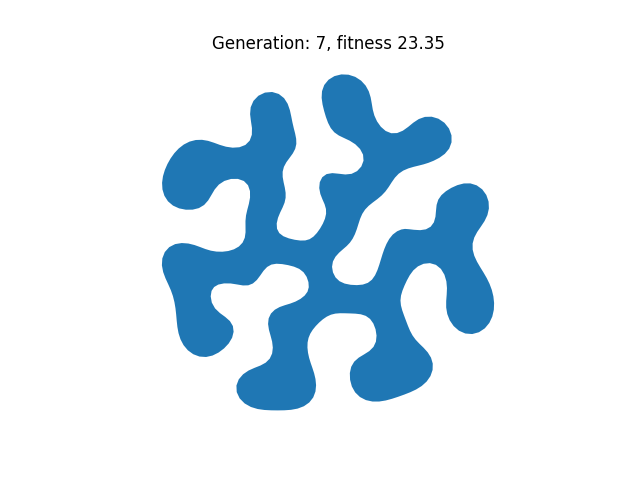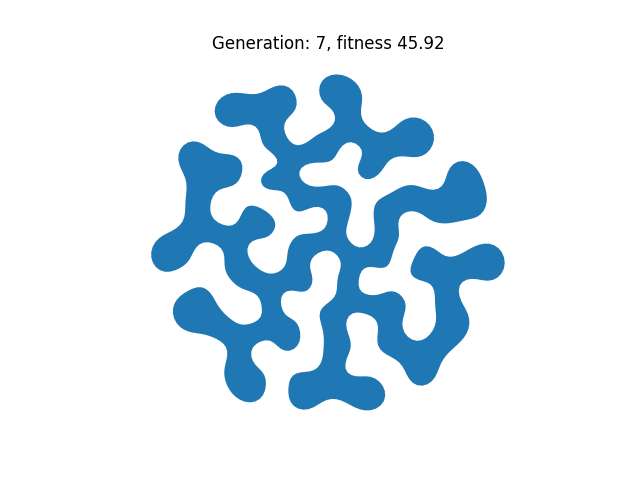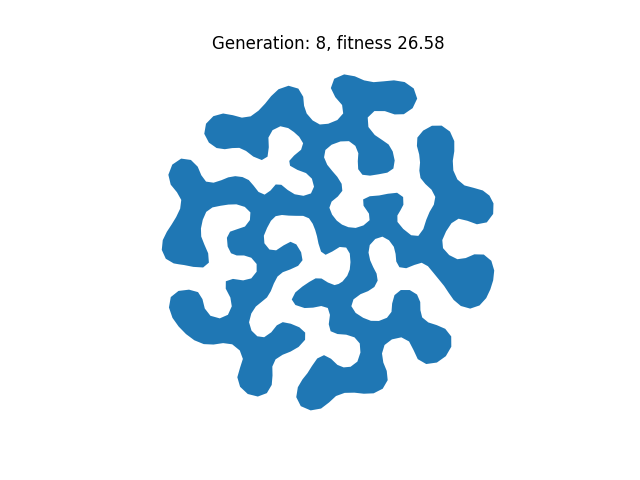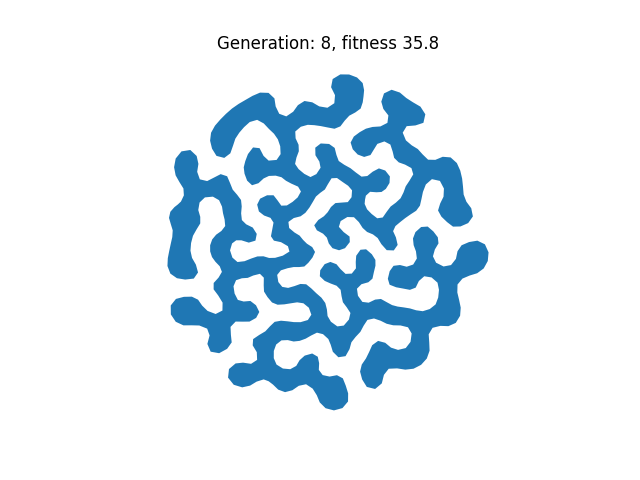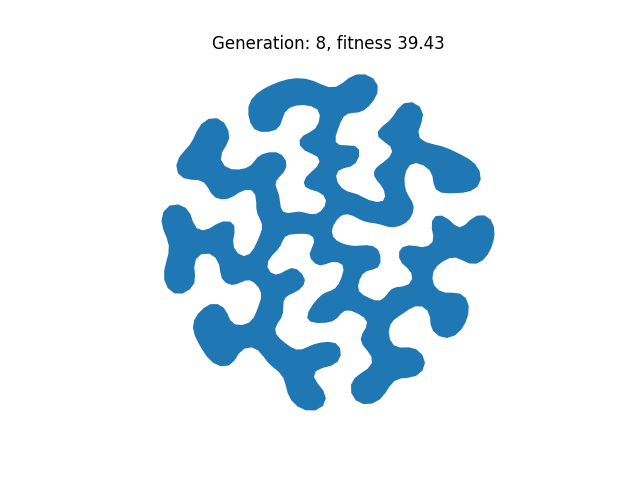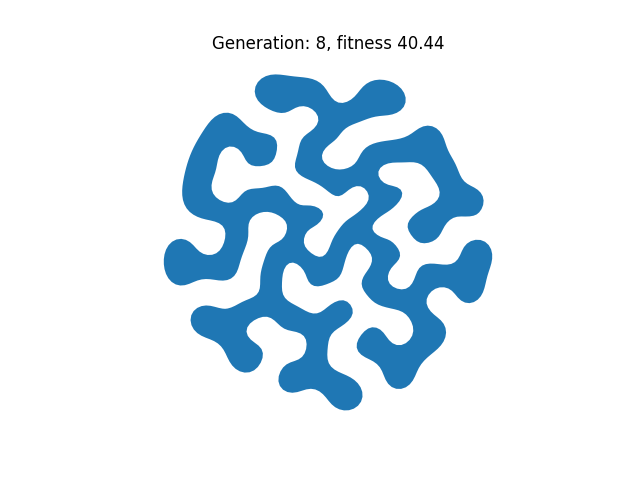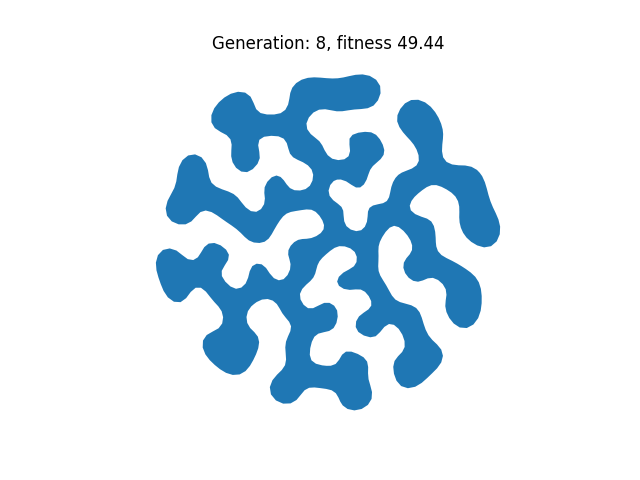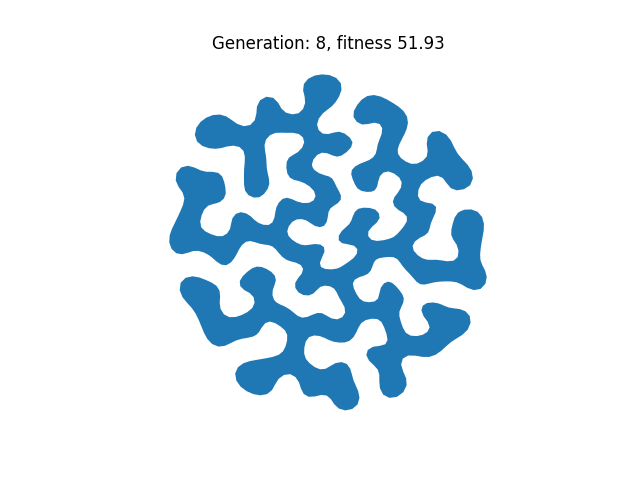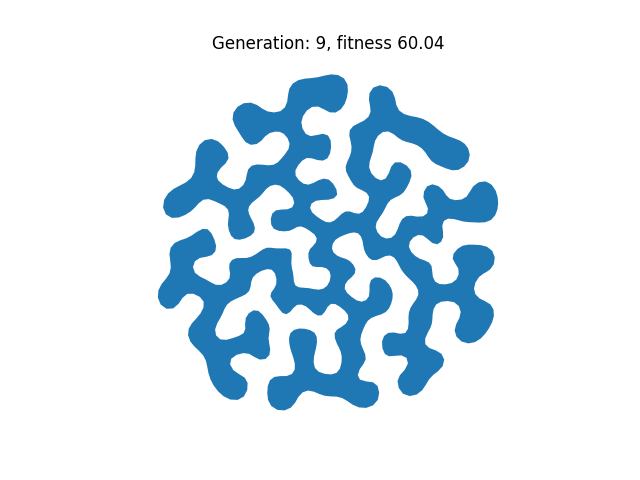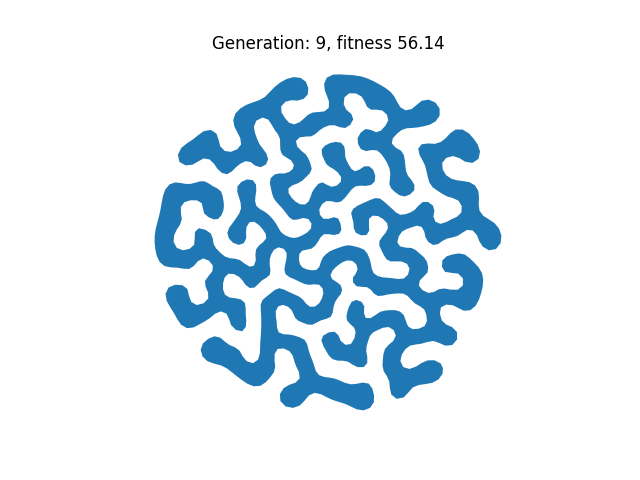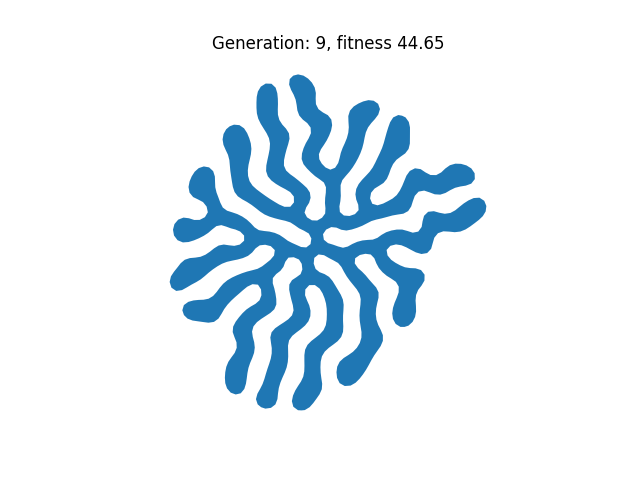# This page illustrates differential growth in 2D combined with genetic algorithms

The shapes below are created by a simple growth process where each vertex (aka point) on the curve is repulsed from all other points but attracted to its two neighbors (i.e. smoothed). Moreover, in each iteration a new vertex is inserted either by splitting the longest edge or a random edge. The images are all examples where this process has run for a number of iterations between 10 and 1200.

The parameters which control the growth are:

• The total number of iterations (10-1200)
• Weight used for smoothing (0.05-0.98)
• Weight used for repulsion (0.01-0.1)
• strategy for inserting vertices (longest edge vs random)

As an experiment, I tried optimizing the parameters using a genetic algorithm. In this experiment the genome is simply a particular configuration of the parameters.

The fitness function used for this evolutionary scheme is the sum for all vertices:

$\sum_{i} \left\{ \begin{array}{rl} \alpha_i^2 & \mathrm{if} \, |\alpha_i|< \pi/6 \\ 0 & \mathrm{otherwise} \end{array}\right.$

where $\alpha_i$ is the turning angle at vertex $i$. This fitness promotes rather undulating shapes, but penalizes sharp corners.

Initially, ten shapes were created by producing ten parameter sets (genomes) at random but within the ranges above.

The fittest shape was then allowed to mate with shapes number 2-7 and the second fittest with shapes 3-6 (in order of decreasing fitness). This produces a total of ten new shapes. The mating process randomly selects each of the four parameters from either parent, producing four new parameters which are then mutated with some probability. The result is a new genome which again consists of four parameters. The growth process is now used to generate a new shape, i.e. phenotype.

In the experiment, ten randomly generated shapes were allowed to evolve for ten generations. The results are shown below. I am not sure how much can be inferred from this extremely simple experiment, but it is somewhat interesting how similar the final shapes are. The fitness function was created to promote shapes like these, so that they arise is not surprising. What is interesting is how few generations it takes from the fittest arising in the population to the near total domination of their genes.